Courses

# Chemistry - 2017 Past Year Paper

## 60 Questions MCQ Test IIT JAM Past Year Papers and Model Test Paper (All Branches) | Chemistry - 2017 Past Year Paper

Description
This mock test of Chemistry - 2017 Past Year Paper for IIT JAM helps you for every IIT JAM entrance exam. This contains 60 Multiple Choice Questions for IIT JAM Chemistry - 2017 Past Year Paper (mcq) to study with solutions a complete question bank. The solved questions answers in this Chemistry - 2017 Past Year Paper quiz give you a good mix of easy questions and tough questions. IIT JAM students definitely take this Chemistry - 2017 Past Year Paper exercise for a better result in the exam. You can find other Chemistry - 2017 Past Year Paper extra questions, long questions & short questions for IIT JAM on EduRev as well by searching above.
QUESTION: 1

Solution:
QUESTION: 2

Solution:
QUESTION: 3

### A yellow precipitate is formed upon addition of aqueous AgNO3 to a solution of :

Solution:
QUESTION: 4

The compounds having C3- axis of symmetry are :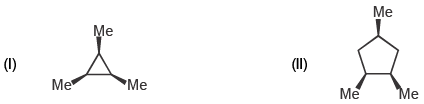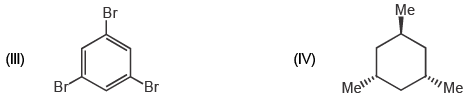Solution:
QUESTION: 5

The correct order of rate of solvolysis for the following compounds is: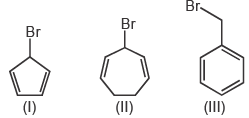Solution:
QUESTION: 6

In the following sequence of reactions, the overall yield (%) of O is :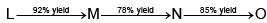Solution:
QUESTION: 7

Catalytic hydrogenation of the following compound produces saturated hydrocarbon(s). The number of stereoisomer(s) formed is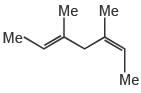Solution:
QUESTION: 8

The number of normal modes of vibration in naphthalene is :

Solution:
QUESTION: 9

The number of degrees of freedom of liquid water in equilibrium with ice is:

Solution:
QUESTION: 10

A straight line having a slope of -ΔUo / R is obtained in a plot between :

Solution:
QUESTION: 11

In a typical conductometric titration of a strong acid with a weak base, the curve resembles:

Solution:
QUESTION: 12

The coordination number of Al in crystalline AICl3 and liquid AICI3, respectively, is :

Solution:
QUESTION: 13

The homogeneous catalyst used in water- gas shift reaction is :

Solution:
QUESTION: 14

Nitrosyl ligand binds to d- metal atoms in linear and bent fashion and behaves, respectively, as:

Solution:
QUESTION: 15

The metal ion (M2+) in the following reaction is :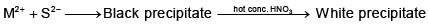Solution:
QUESTION: 16

The correct order of wavelength of absorption (λmax) of the Cr-complexes is (en-ethylenediamine)

Solution:
QUESTION: 17

The correct order of enthalpy of hydration for the transition metal ions is :

Solution:
QUESTION: 18

Which of the following is/are the S-enantiomer of alanine?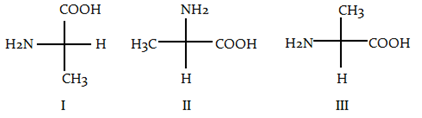Solution:

1 and 3 have an S configuration, but 2 has an R configuration. The priority of the substituents on the chirality center of alanine is: -NH2 > -COOH > -CH3 > -H.

QUESTION: 19

The number of proton NMR signals for the compounds P and Q, respectively is :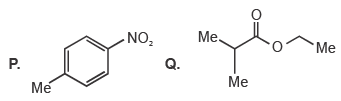Solution:
QUESTION: 20

The correct set of reagents for the following conversion is :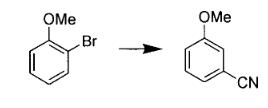Solution:
QUESTION: 21

The product R in the following reaction is :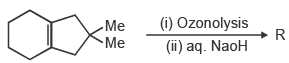Solution:
QUESTION: 22

The major product S of the following reaction is :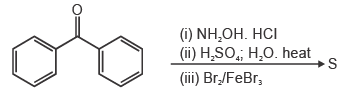Solution:
QUESTION: 23

In the following reaction, the major product T is :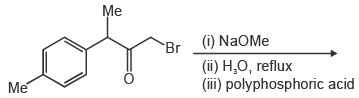Solution:
QUESTION: 24

The following conversion is carried out using :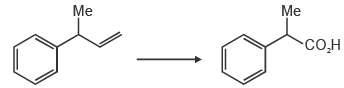Solution:
QUESTION: 25

In the following reactions, the major product E and F, respectively, are :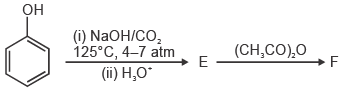Solution:
QUESTION: 26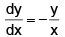is a differential equation for a/an :

Solution:
QUESTION: 27

Value of the given determinant is :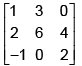Solution:
QUESTION: 28

Ionization energy of hydrogen atom in ground state is 13.6 eV. The energy released (in eV) for third member of Balmer series is :

Solution:
QUESTION: 29

For a first order reaction A(g) → 2B(g) + C(g), the rate constant in terms of initial pressure (p0) and pressure at time t(pt), is given by :

Solution:
QUESTION: 30

For a particle in one- dimensional box of length L with potential energy V(x) = 0 and L > x > 0 and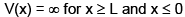an acceptable wave function consistent with the boundary conditions is (a, b, c and d are constants)

Solution:
*Multiple options can be correct
QUESTION: 31

The “heme” containing protein(s) is/are :

Solution:
*Multiple options can be correct
QUESTION: 32

Among the following, the species having see- saw shape is/are :

Solution:
*Multiple options can be correct
QUESTION: 33

The indicator(s) appropriate for the determination of end point in the titration fo a weak acid with a strong base is/are :

Solution:
*Multiple options can be correct
QUESTION: 34

Jahn- Taller distortion is/are observed in octahedral complexes with d- electron configuration of :

Solution:
*Multiple options can be correct
QUESTION: 35

Among the following the correct statement(s) is/are :

Solution:
*Multiple options can be correct
QUESTION: 36

The INCORRECT statement(s) among the following is/are:

Solution:
QUESTION: 37

The following conversion(s) is/are example(s) of :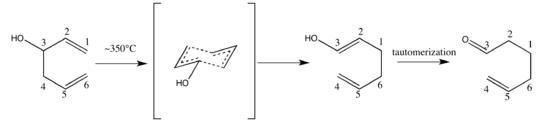Solution:
*Multiple options can be correct
QUESTION: 38

IR active molecule(s) is/are:

Solution:
*Multiple options can be correct
QUESTION: 39

Intensity variable(s) is/are :

Solution:
*Multiple options can be correct
QUESTION: 40

Wave nature of electromagnetic is observed in :

Solution:
*Answer can only contain numeric values
QUESTION: 41

The number of isometric structures of di- substituted borazine (B3N3H4X2) is __________.

Solution:
*Answer can only contain numeric values
QUESTION: 42

The number of S- S bond(s) in tetrathionate ion is ___________.

Solution: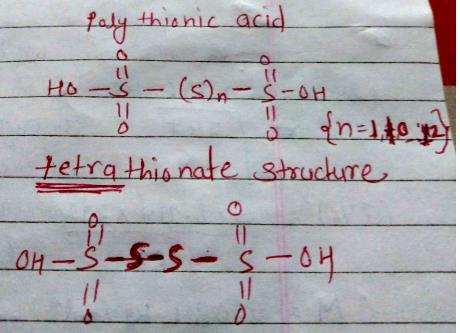Hence 3 is the correct answer.

*Answer can only contain numeric values
QUESTION: 43

The number of unpaired electron(s) is K2NiF6 is _________ .

Solution:
*Answer can only contain numeric values
QUESTION: 44

The number of reducing sugars among the following is __________.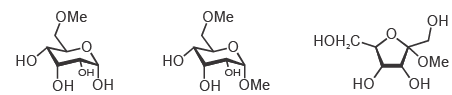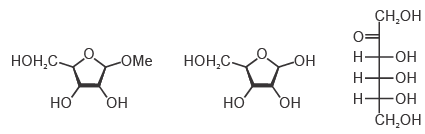Solution:
*Answer can only contain numeric values
QUESTION: 45

The maximum number of dipeptides that could be obtained by reaction of phenylalanine with Leucine is ________.

Solution:
*Answer can only contain numeric values
QUESTION: 46

Among the following, the number of aromatic compound(s) is ___________.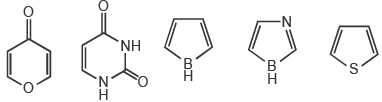Solution:
*Answer can only contain numeric values
QUESTION: 47

At an operating frequency of 350 MHz, the shift (in Hz) of resonance from TMS (tetramethylsilane) of a proton with chemical shift of 2 ppm is ___________.

Solution:
*Answer can only contain numeric values
QUESTION: 48

At 298 K and 1 atm, the molar enthalpies of combustion of Cyclopropane and propene are – 2091 kJ mol–1 and –2058 kJ mol–1, respectively. The enthalpy change (in kJ mol–1) for the conversion of one mole of propene to one mole of Cyclopropane is _________.

Solution:
*Answer can only contain numeric values
QUESTION: 49

For a cell reaction Pb(s) + Hg2Cl2(s) → PbCl2(s) + 2Hg(I),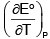is 1.45 × 10–1 VK–1. The
entropy change (in J mol–1 K–1) for the reaction is [Given 1 F = 96500 C mol–1] _______.

Solution:
*Answer can only contain numeric values
QUESTION: 50

For a reaction 2A + B → C + D, if rate of consumption of A is 0.1 mol L–1 s–1, the rate of production of C (in mol L–1 s–1) is ___________.

Solution:
*Answer can only contain numeric values
QUESTION: 51

The standard reduction potentials of Ce4+/Ce3+ and Fe3+/Fe2+ are 1.44 and 0.77 V, respectively. The log10K (K is the equilibrium constant) value for the following reaction is ________. (Given RT/F = 0.0257).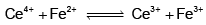Solution:
*Answer can only contain numeric values
QUESTION: 52

A radioactive element undergoes 80% radioactive decay in 300 min. The half- life for this species in minutes is _________.

Solution:
*Answer can only contain numeric values
QUESTION: 53

Silver crystallizes in face- centered cubic lattice. The lattice parameter of silver (in picometer) is ______.
[Given : Avogadro’s number = 6.023 × 1023 mol–1, molar mass of silver = 107.87 g mol–1 and density of crystal = 10.5 g cm–3]

Solution:
*Answer can only contain numeric values
QUESTION: 54

The amount of bromine (atomic wt. = 80) required (in gram) for the estimation of 42.3 g of phenol (molecule wt. = 94 g mol–1) is ____________.

Solution:
*Answer can only contain numeric values
QUESTION: 55

The total number of pair of enantiomers possible with molecular formula C5H12O is ________.

Solution:
*Answer can only contain numeric values
QUESTION: 56

In 200 g of water 0.01 mole of NaCI and 0.02 mole of sucrose are dissolved. Assuming solution to be ideal, the depression in freezing point of water (in oC) will be ________. (final answer should be rounded off to two decimal places) [Given : Kf(H2O) = 1.86 K kg mol–1]

Solution:
*Answer can only contain numeric values
QUESTION: 57

The adsorption of a gas follows the Langmuir isotherm with K = 1.25 KPa–1 at 25°C. The pressure (in Pa) at which surface coverage is 0.2 is ________.

Solution:
*Answer can only contain numeric values
QUESTION: 58

The separation fo 123 planes (in nm) in an orthorhombic cell with a = 0.25 nm, b = 0.5 nm and c = 0.75 nm is ___________.
(final answer should be rounded off to two decimal places)

Solution:
*Answer can only contain numeric values
QUESTION: 59

A vessel contains a mixture of H2 and N2 gas. The density of this gas mixture is 0.2 g L–1 at 300 K and 1 atm. Assuming that both the gases behave ideally, the mole fraction of N2(g) in the vessel is __________.
(final answer should be rounded off to two decimal places)
[Given: R = 0.082 L atm mol–1 K–1, atomic wt. of hydrogen = 1.0 and atomic wt. of nitrogen = 14.0]

Solution:
*Answer can only contain numeric values
QUESTION: 60

Consider an isothermal reversible compression of one mole of an ideal gas in which the pressure of the system is increased from 5 atm to 30 atm at 300 K. The entropy change of the surroundings (in JK–1) is __________.
(final answer should be rounded off to two decimal places)
[Given: R = 8.314 J mol–1 K–1]

Solution: